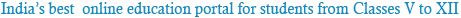### NCERT Solutions for Class 9th Science: Chapter 3: Atoms and Molecules

Question 1:
In a reaction, 5.3 g of sodium carbonate reacted with 6 g of ethanoic acid. The products were 2.2 g of carbon dioxide, 0.9 g water and 8.2 g of sodium ethanoate. Show that these observations are in agreement with the law of conservation of mass. Sodium carbonate + ethanoic acid → sodium ethanoate + carbon dioxide + water
In the given reaction, sodium carbonate reacts with ethanoic acid to produce sodium ethanoate, carbon dioxide, and water.Question 2:
Hydrogen and oxygen combine in the ratio of 1:8 by mass to form water. What mass of oxygen gas would be required to react completely with 3 g of hydrogen gas?
It is given that the ratio of hydrogen and oxygen by mass to form water is 1:8. Then, the mass of oxygen gas required to react completely with 1 g of hydrogen gas is 8 g. Therefore, the mass of oxygen gas required to react completely with 3 g of hydrogen gas is 8 × 3 g = 24 g.

Question 3:
Which postulate of Dalton’s atomic theory is the result of the law of conservation of mass?
The postulate of Dalton’s atomic theory which is a result of the law of conservation of mass is: Atoms are indivisible particles, which can neither be created nor destroyed in a chemical reaction.

Question 4:
Which postulate of Dalton’s atomic theory can explain the law of definite proportions?
The postulate of Dalton’s atomic theory which can explain the law of definite proportion is:
The relative number and kind of atoms in a given compound remains constant.

Question 1:
Define atomic mass unit.
Mass unit equal to exactly one-twelfth the mass of one atom of carbon-12 is called one atomic mass unit. It is written as ‘u’.

Question 2:
Why is it not possible to see an atom with naked eyes?
Thesize of an atom is so small that it is not possible to see it with naked eyes. Also, the atom of an element does not exist independently.Answer: The chemical formula of a compound means the symbolic representation of the composition of a compound. From the chemical formula of a compound, we can know the number and kinds of atoms of different elements that constitute the compound. For example, from the chemical formula CO2 of carbon dioxide, we come to know that one carbon atom and two oxygen atoms are chemically bonded together to form one molecule of the compound, carbon dioxide.

Question 4:
How many atoms are present in a
(i) H2S molecule and
(ii) PO4 3− ion?
(i) In an H2S molecule, three atoms are present; two of hydrogen and one of sulphur.
(ii) In a PO4 3− ion, five atoms are present; one of phosphorus and four of oxygen.Powered by: iVerticals Web Technology Pvt Ltd